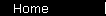# Compound Annual Growth Rate (Annualized Return)

A problem with talking about average investment returns is that there is real ambiguity about what people mean by "average". For example, if you had an investment that went up 100% one year and then came down 50% the next, you certainly wouldn't say that you had an average return of 25% = (100% - 50%)/2, because your principal is back where it started: your real annualized gain is zero.

In this example, the 25% is the simple average, or "arithmetic mean". The zero percent that you really got is the "geometric mean", also called the "annualized return", or the CAGR for Compound Annual Growth Rate.

Volatile investments are frequently stated in terms of the simple average, rather than the CAGR that you actually get. (Bad news: the CAGR is smaller.)

## CAGR of the Stock Market

This calculator lets you find the annualized growth rate of the S&P 500 over the date range you specify; you'll find that the CAGR is usually about a percent or two less than the simple average.

Year and Return (%)

Date Range

Jan 1 to Dec 31

 "Average" return: % Annualized return (= True CAGR): % Standard Deviation: % \$1.00 grew to: \$

 S&P 500 returns (dividends included): Robert Shiller and Yahoo! Finance (Last year's data subject to revision.)

The most significant pattern is this:

 Over the very long run, the stock market has had an inflation-adjusted annualized return rate of between six and seven percent.

Another pattern: while stocks have certainly beaten inflation over the long run, they've done poorly within the high-inflation periods themselves: try the inflation-adjusted returns for 1916-1918, 1946-1947, and 1973-1981.

## CAGR Approximation

There is a formula that lets you estimate the CAGR if you already know the simple average and the standard deviation:

(1 + rave)2  -  StdDev2   =   (1 + CAGR)2

In the example at the top of the page, the simple average is 25% and the standard deviation is 75% (since the data points of +100 and -50 lie at a distance of 75 away from the simple average); in this case the estimate gives the exact answer of zero for the CAGR:

 rave: %         StdDev: %         CAGR: % (Approximation. You can change any of these inputs.)

In the case of stock market returns, if you plug in the results of the first calculator you'll find that the approximation isn't exact, but it's still pretty good.Stock Market Returns

 Also See...